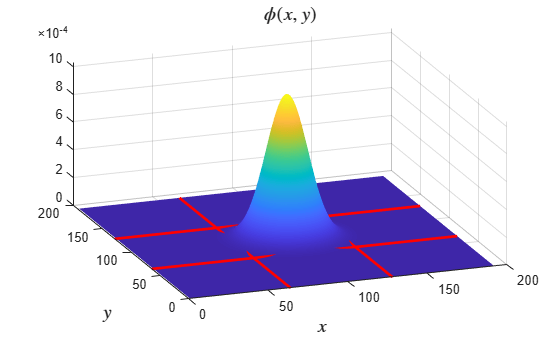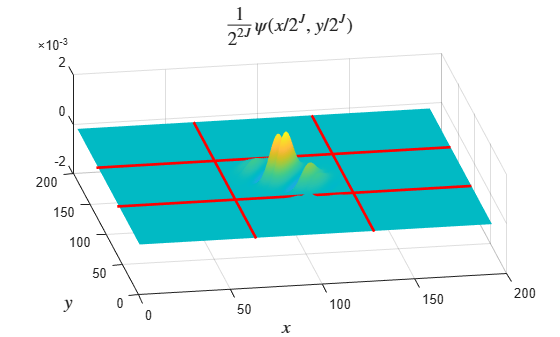waveletScattering2

Wavelet image scattering

Description

Use the waveletScattering2 object to create a network for a wavelet image scattering decomposition using complex-valued 2-D Morlet wavelets.

Creation

Description

example

sf = waveletScattering2 creates a network for a wavelet image scattering decomposition with two complex-valued 2-D Morlet filter banks and isotropic scale invariance. Both filter banks have quality factors of one wavelet per octave. There are six rotations linearly spaced between 0 and π radians for each wavelet filter. By default, waveletScattering2 assumes an image input size of 128-by-128. The scale invariance is 64.

sf = waveletScattering2(Name,Value) creates a network for wavelet image scattering with properties specified by one or more Name,Value pair arguments. Properties can be specified in any order as Name1,Value1,...,NameN,ValueN. Enclose each property name in single quotes (' ') or double quotes (" ").

Note

With the exceptions of OptimizePath and OversamplingFactor, you cannot change a property value of an existing scattering network. For example, if you create a network sf with ImageSize set to [256 256], you cannot assign a different ImageSize to sf.

Properties

expand all

Image size for wavelet image scattering network, specified as a two-element integer-valued vector [numrows numcolumns]. Images must be at least 10-by-10.

If your input is an RGB image, you do not have to specify the third dimension. waveletScattering2 only supports color images where the size of the third dimension is 3.

Example: sf = waveletScattering2('ImageSize',[100 200]) creates a network for 100-by-200 images and 100-by-200-by-3 color images.

Scattering transform invariance scale, specified as a positive scalar. InvarianceScale specifies the spatial support in the row and column dimensions of the scaling filter. InvarianceScale cannot exceed the minimum size of the row and column dimensions of the image.

By default, InvarianceScale is one-half the minimum of the row and column sizes of the image rounded to the nearest integer.

Example: sf = waveletScattering2('ImageSize',[101 200]) creates a framework with InvarianceScale equal to 51.

Number of rotations per wavelet per filter bank in the scattering network, specified as an integer-valued vector. Specify one integer less than or equal to 12 for each filter bank in the scattering network.

For each wavelet in each filter bank, there are NumRotations linearly spaced angles between 0 and π radians. The wavelet is rotated in a clockwise direction. The length of the vector specified in NumRotations must equal the length of the vector specified in QualityFactors.

Example: sf = waveletScattering2('NumRotations',[7 5]) creates a network with seven rotations per wavelet in the first filter bank and five rotations per wavelet in the second filter bank.

Note

The 2-D wavelet scattering network is constructed by rotating the 2-D Morlet wavelets in a clockwise direction. The opposite convention is used in the Image Processing Toolbox™. Creating a Gabor filter bank to apply to an image involves rotating the Gabor filter in a counter-clockwise direction. See Slant Parameter, and gabor (Image Processing Toolbox) in the Image Processing Toolbox.

Scattering filter bank quality factors, specified as an integer-valued vector. The quality factor is the number of wavelet filters per octave. The number of wavelet filter banks in the scattering network is equal to the number of elements in QualityFactors. Valid quality factors are integers less than or equal to 4. If QualityFactors is specified as a vector, the elements of QualityFactors must be nonincreasing.

The length of the vector specified in QualityFactors must equal the length of the vector specified in NumRotations.

Example: sf = waveletScattering2('QualityFactors',[2 1])

Precision of scattering coefficients and filters:

• 'single' — Single precision

• 'double' — Double precision

Note

• All calculations involving the wavelet scattering network are carried out in Precision.

• The precision of the output of the scatteringTransform function does not exceed the precision of the waveletScattering2 object.

Oversampling factor, specified as a nonnegative integer or Inf. The factor specifies how much the image scattering coefficients are oversampled with respect to the critically downsampled values. The oversampling factor is on a log2 scale. For example, if sf = waveletScattering2('OversamplingFactor',1), the scattering transform returns 21-by-21-by-P as many coefficients for each scattering path with respect to the critically sampled number. You can use coefficientSize to determine the number of coefficients obtained for a scattering network. By default, OversamplingFactor is set to 0, which corresponds to critically downsampling the coefficients.

If you specify an oversampling factor that would result in an output image size larger than the input, the output size is truncated to the size of the input image. You can also specify the OversamplingFactor as Inf, which provides a fully undecimated scattering transform where each scattering path contains coefficient matrices equal in size to the input image.

Due to the computational complexity of the scattering transform, the recommended setting for the OversamplingFactor property is 0, 1, or 2. Values of 1 and 2 indicate a 21-by-21-by-P and a 22-by-22-by-P increase in the number of scattering coefficients per path, respectively.

Example: sf.OversamplingFactor = 1 sets the OversamplingFactor property of an existing network to 1.

Optimize scattering transform logical, which determines whether the scattering transform reduces the number of scattering paths to compute based on a bandwidth consideration.

When OptimizePath is set to true, a scattering path is computed only if the bandwidth of the parent node overlaps significantly with the bandwidth of the child node. 'Significant' in this context is defined as follows: for a quality factor of 1, 1/2 the 3-dB bandwidth of the child node is subtracted from the child node's wavelet center frequency. If that value is less than the 3-dB bandwidth of the parent, the scattering path is computed. For quality factors greater than 1, significant overlap is defined to be an overlap between the center frequency of the child minus the child's 3-dB bandwidth. If that overlaps with the 3-dB bandwidth of the parent, the scattering path is computed.

You can use paths to determine which and how many scattering paths are computed. OptimizePath generally results in computational savings in the second and subsequent filter banks only when the quality factors are equal in each filter bank.

Example: sf.OptimizePath = false sets the OptimizePath property of an existing network to false.

Object Functions

 scatteringTransform Wavelet 2-D scattering transform featureMatrix Image scattering feature matrix log Natural logarithm of 2-D scattering transform filterbank Wavelet and scaling filters littlewoodPaleySum Littlewood-Paley sum coefficientSize Size of image scattering coefficients numorders Number of scattering orders numfilterbanks Number of scattering filter banks paths Scattering paths

Examples

collapse all

Create a wavelet image scattering network with default settings. The default image size is 128-by-128, and the default invariance scale is 64.

sf = waveletScattering2
sf =
waveletScattering2 with properties:

ImageSize: [128 128]
InvarianceScale: 64
NumRotations: [6 6]
QualityFactors: [1 1]
Precision: 'single'
OversamplingFactor: 0
OptimizePath: 1

Use the filterbank function to obtain the Fourier transform of the scaling function, the wavelet filters, and the center spatial frequencies of the wavelet filters.

[phif,psif,f] = filterbank(sf);

The invariance scale gives the width in the x- and y-directions of the 2-D Gaussian scaling function. To confirm the scaling function has the expected spatial width, first take the inverse Fourier transform of phif. Use the helper function helperPlotPhiSurface to plot the scaling function with the extent of the invariance scale in both x and y designated. The source code for helperPlotPhiSurface is provided in the appendix at the end of this example.

phi = ifftshift(ifft2(phif));
figure
helperPlotPhiSurface(sf,phi)The scaling function is larger than 128-by-128 because it has been padded to avoid edge effects.

Extract the Fourier transform of the coarsest scale wavelet in the second filter bank and take its inverse Fourier transform. Use helperPlotPsiSurface to plot the real and imaginary parts of the wavelet and confirm the spatial extent of the coarsest scale wavelet does not exceed the invariance scale. Similar to the scaling function, the wavelet has been padded to avoid edge effects. The source code for helperPlotPsiSurface is provided in the appendix at the end of this example.

psiF = psif{2}(:,:,end);
psiL = ifftshift(ifft2(psiF));
figure
helperPlotPsiSurface(sf,psiL)Appendix

The following helper functions are used in this example.

helperPlotPhiSurface

function helperPlotPhiSurface(scatFrame,data)
halfscale = scatFrame.InvarianceScale/2;
surf(data)
view(-20,35)
Ysize = size(data,1);
Xsize = size(data,2);
Ycenter = Ysize/2;
Xcenter = Xsize/2;
hold on
plot([Xcenter-halfscale Xcenter-halfscale],[0 Ysize],'r','LineWidth',2);
plot([Xcenter+halfscale Xcenter+halfscale],[0 Ysize],'r','LineWidth',2);
plot([0 Xsize],[Ycenter-halfscale Ycenter-halfscale],'r','LineWidth',2);
plot([0 Xsize],[Ycenter+halfscale Ycenter+halfscale],'r','LineWidth',2);
title('$\phi(x,y)$','FontSize',14,'Interpreter','Latex');
xlabel('$x$','FontSize',14,'Interpreter','Latex')
ylabel('$y$','FontSize',14,'Interpreter','Latex')
end

helperPlotPsiSurface

function helperPlotPsiSurface(scatFrame,data)
halfscale = scatFrame.InvarianceScale/2;
Ysize = size(data,1);
Xsize = size(data,2);
Ycenter = Ysize/2;
Xcenter = Xsize/2;
surf(real(data))
view(-5,13)
hold on
surf(imag(data))
plot([Xcenter-halfscale Xcenter-halfscale],[0 Ysize],'r','LineWidth',2);
plot([Xcenter+halfscale Xcenter+halfscale],[0 Ysize],'r','LineWidth',2);
plot([0 Xsize],[Ycenter-halfscale Ycenter-halfscale],'r','LineWidth',2);
plot([0 Xsize],[Ycenter+halfscale Ycenter+halfscale],'r','LineWidth',2);
title('$\frac{1}{2^{2J}}\psi(x/2^J,y/2^J)$','FontSize',14,...
'Interpreter','Latex');
xlabel('$x$','FontSize',14,'Interpreter','Latex')
ylabel('$y$','FontSize',14,'Interpreter','Latex')
view(-10,51)
end

 Bruna, J., and S. Mallat. "Invariant Scattering Convolution Networks." IEEE Transactions on Pattern Analysis and Machine Intelligence. Vol. 35, Number 8, 2013, pp. 1872–1886.

 Sifre, L., and S. Mallat. "Rigid-Motion Scattering for Texture Classification". arXiv preprint. 2014, pp. 1–19. https://arxiv.org/abs/1403.1687.

 Sifre, L., and S. Mallat. "Rotation, scaling and deformation invariant scattering for texture discrimination." 2013 IEEE Conference on Computer Vision and Pattern Recognition. 2013, pp 1233–1240.

Wavelet Toolbox DocumentationGet trial now